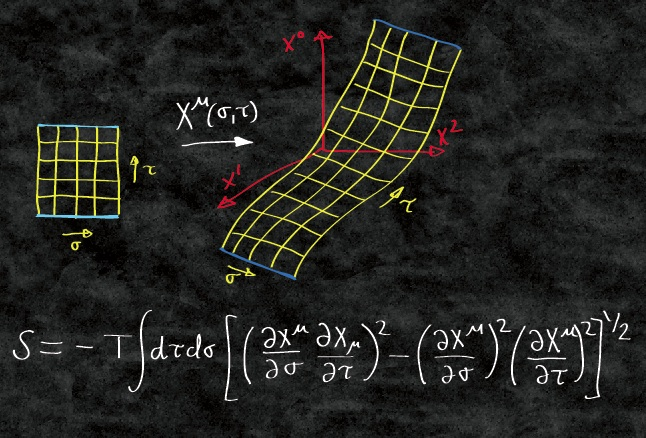# Here is a glimpse of my daily experience## Realized Garch model with dynamical conditional correlation

Study of Garch model with realized variance and study the dynamic conditional correlation model on 6 stock stock data.

## My cute son’s photos before he turns to one month

My son turns to one month and he is so cute. He has a name now : Xuanyi Ben Fan (范本轩逸).

## Travel Experience to Hawaii

Travel Plan I have always been dreaming of traveling to Hawaii and enjoy the beach and sunshine. This spring break we got cheap tickets and make our plan to...

My son is born !

## EM Algorithm for Linear Clusters

Here is an interesting situation occurred to me. In a scatter plot, it is clear that there are two straight lines and each line is contanminated by some nois...

## Some FPGA basic element design and Vivado code

I am doing a service task for CERN on a hardware project. I learned something on FPGA and some basic vivado code and glad to share here.

## Introduction on how to write an Athena(ATLAS) package for release22

I am writing this post is because I recently met some problem of writing an Athena Algorithm for my research. I am using a simple code to get lepton n-tuples...

## Some Properties of Linear Regression

If we regress $X$ on $Y$ and get $\beta$ as the slope and if we regress $Y$ on $X$ and get slope $\beta’$. What is the product of $\beta$ and $\beta’$

## Interesting Statsitcal Problems in Interviews

Problem 1 If we have $n$ dice draws with a dice that has $k$ sides. Let $X_i$ to be the random variables that describes the number of times that the dice fac...